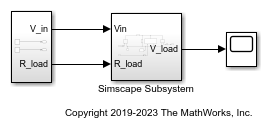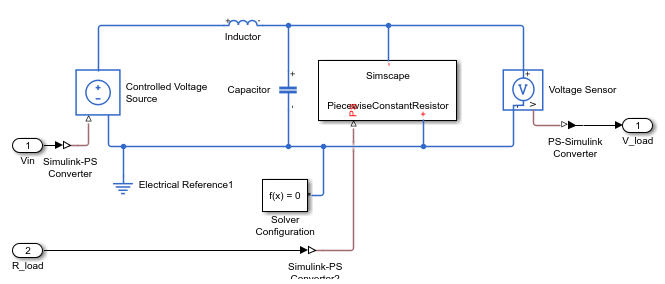Replacing Piecewise-Constant Resistor

This example shows how to convert a model that is nonlinear due to a Piecewise-Constant Resistor into a switched linear model making it compatible with Simscape to HDL Workflow.

Introduction

Simscape to HDL Workflow supports conversion of Simscape switched linear models to functionally-equivalent Simulink models that are compatible for HDL code generation. This example uses Piecewise-Constant Resistor which is event based and must be replaced with equivalent switched linear components. Specifically the Piecewise-Constant Resistor contains events that are not supported by the Simscape HDL Workflow Advisor.

Open The Simscape™ Model. In the MATLAB® command prompt, enter:

nonlinearModel = 'sschdlexVariableResistorExample';
open_system([nonlinearModel,'/Simscape Subsystem'])This model is an RLC circuit with a Piecewise-Constant Resistor acting as the resistor or 'load'. For the Piecewise-Constant Resistor, the relationship between voltage V and current I is V=I*R where R is the numerical value presented at the physical signal port R.

To ensure a positive value for the resistance, any value below 1e-6 is replaced by 1e-6. This resistor is Piecewise-Constant because the resistance only changes when the input value differs from the current resistance by more than a set tolerance. Thus, a continuously changing input would be converted to a discrete set of resistances.

In this model the signal going into the Piecewise-Constant Resistor is a step function that changes from 2 to 3 at t=0.1 thus changing the load resistance from 2to 3.To open the Simscape HDL Workflow Advisor at the command-line, enter: sschdladvisor(nonlinearModel)

Run the workflow to the Get state-space parameters task. This task fails because of the presence of the Piecewise-Constant Resistor.Replace Piecewise-Constant Resistor with switches and constant resistors.

To convert this model to an equivalent switched linear model, replace the Piecewise-Constant Resistor with a set of switches and resistors for each desired value. To open the switched linear version in the MATLAB® command prompt, enter:

switchedLinearModel = 'sschdlexVariableResistorSwitchedLinearExample';
open_system(switchedLinearModel)The Piecewise-Constant Resistor has been replaced by a resistor and switch for each desired resistance. To recreate the behavior of a load resistance that changes from 2to 3at t=0.1 two resistors are used, one with a resistance of 2and the other with a resistance of 3. By closing and opening the switches the load resistance switches from 2to 3.

Controlling the Switches

The switches must be turned on and off to provide the correct load resistance. To view the control signals for the switches in the MATLAB® command prompt, enter:

open_system([switchedLinearModel,'/Input'])To achieve the correct resistance, create two step functions. One to open the switch in series with the 2resistor at t=0.1 and another to close the switch in series with the 3resistor at the same time.

Now that the Piecewise-Constant Resistor has been replaced with switched linear components run the Simscape HDL Workflow Advisor and see that all the tasks run to completion.By changing the Piecewise-Constant Resistor to a number of specified resistors that switch on and off the model has been changed to a form that is compatible with the Simscape to HDL workflow.